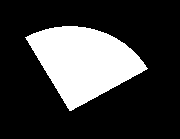# sector() function in C

The header file graphics.h contains sector() function which draws and fills an elliptical pie slice with (x, y) as center, (s_angle, e_angle) as starting and ending angle and (x_radius, y_radius) as x and y radius of sector.
Syntax :

```void sector(int x, int y, int s_angle,

where,
(x, y) is center of the sector.
(s_angle, e_angle) are starting
and ending angles.
```

Examples :

```Input : x = 200, y = 200, s_angle = 0,
e_angle = 150, x_radius = 50,
Output :Input : x = 200, y = 200, s_angle = 30,
e_angle = 120, x_radius = 90,
Output :```

Below is the implementation of sector() function :

 `// C Implementation for sector() ` `#include ` ` `  `// driver code ` `int` `main() ` `{ ` `    ``// gm is Graphics mode which is ` `    ``// a computer display mode that ` `    ``// generates image using pixels. ` `    ``// DETECT is a macro defined in ` `    ``// "graphics.h" header file ` `    ``int` `gd = DETECT, gm; ` ` `  `    ``// initgraph initializes the ` `    ``// graphics system by loading a ` `    ``// graphics driver from disk ` `    ``initgraph(&gd, &gm, ``""``); ` ` `  `    ``// sector function ` `    ``sector(200, 200, 0, 150, 50, 65); ` ` `  `    ``getch(); ` ` `  `    ``// closegraph function closes the ` `    ``// graphics mode and deallocates ` `    ``// all memory allocated by ` `    ``// graphics system . ` `    ``closegraph(); ` ` `  `    ``return` `0; ` `} `

Output :

``````

My Personal Notes arrow_drop_upIn love with a semicolon because sometimes i miss it so badly)

If you like GeeksforGeeks and would like to contribute, you can also write an article using contribute.geeksforgeeks.org or mail your article to contribute@geeksforgeeks.org. See your article appearing on the GeeksforGeeks main page and help other Geeks.

Please Improve this article if you find anything incorrect by clicking on the "Improve Article" button below.

Improved By : Akanksha_Rai

Article Tags :
Practice Tags :

Be the First to upvote.

Please write to us at contribute@geeksforgeeks.org to report any issue with the above content.